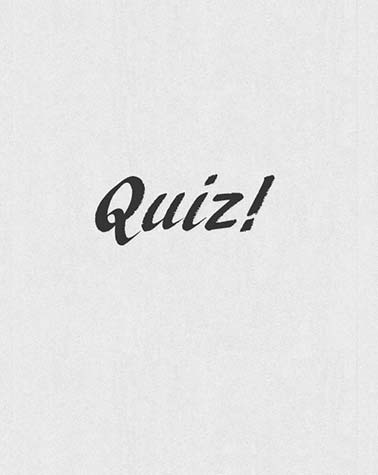# High Efficiency Power Amplifiers

30 Questions | Total Attempts: 41Settings• 1.
The conditions for unconditional stability of a transistor are
• A.

K>1, B>0 and µ>1

• B.

K<1, B>0 and µ>1

• C.

K>1, B>0 and µ<1

• D.

None of the above

• 2.
The main advantage of switched mode PA is
• A.

Bandwidth improvement

• B.

Linearity improvement

• C.

Efficiency improvement

• D.

Gain improvement

• 3.
The harmonic load conditions of Class F PA are
• A.

Z(2f)=0, Z(3f)=Infinity

• B.

Z(2f)=0, Z(3f)=0

• C.

Z(2f)= Infinity , Z(3f)= Infinity

• D.

Z(2f)= Infinity , Z(3f)=0

• 4.
The second harmonic load in class E/F2 mode is
• A.

Short circuit

• B.

Open circuit

• C.

Capacitive

• D.

Inductive

• 5.
In Class B power amplifier mode which of the components are short circuit
• A.

Even harmonics

• B.

Odd harmonics

• C.

All harmonics

• D.

None of the above

• 6.
The small signal gain is lowest in the case of
• A.

Class A

• B.

Class B

• C.

Class C

• D.

Class AB

• 7.
Among the following, the frequency component that comparatively spreads widely on smith chart is
• A.

Fundamental component

• B.

Second harmonic

• C.

Third harmonic

• D.

None

• 8.
Continuous Class J PA is used to improve
• A.

Bandwidth

• B.

Efficiency

• C.

Bandwidth and Efficiency

• D.

Linearity

• 9.
Which matching condition can be used for the design of PA?
• A.

• B.

• C.

Waveform Engineering

• D.

All of the above

• 10.
Centre of the Smith chart represents
• A.

Short

• B.

Open

• C.

• D.

None of the above

• 11.
The theoretical maximum drain efficiency of 100% can be achieved if
• A.

Power dissipation is zero

• B.

Harmonic power is zero

• C.

Harmonic power and power dissipation are zero

• D.

Fundamental and harmonic powers are zero

• 12.
In Class F power amplifier transistor operates as
• A.

Switch

• B.

Voltage source

• C.

Current source

• D.

All odd harmonics

• 13.
Which of the following power amplifier mode can achieve theoretical 100% drain efficiency with minimum number of harmonics
• A.

Class AB

• B.

Class F

• C.

Class J

• D.

Class E

• 14.
The transistor is operated as a switch in
• A.

Class F-1

• B.

Class E

• C.

Class J

• D.

Class F

• 15.
What are the conditions to ensure the lossless operation when voltage is applied across the switch?
• A.

Bias condition

• B.

ZVDS

• C.

ZVS

• D.

ZVS and ZVDS

• 16.
The load conditions of waveform engineered power amplifiers are defined at
• A.

Current source reference plane

• B.

Package plane

• C.

Parasitic plane

• D.

None of the above

• 17.
The second harmonic load in continuous class B/J is
• A.

Complex

• B.

Purely resistive

• C.

Short circuit

• D.

Purely reactive

• 18.
The continuous class E/F mode operates as conventional class E mode when φ1 is
• A.

43.3⁰

• B.

57.5⁰

• C.

78⁰

• D.

90⁰

• 19.
Doherty power amplifier is used to improve
• A.

Output power at saturation

• B.

Efficiency at saturation

• C.

Efficiency at back-off

• D.

Output power at back-off

• 20.
LINC stands for
• A.

Low-noise Amplification with Nonlinear Components

• B.

Linear Amplification with Nonlinear Components

• C.

Low-noise Amplification with Nominal Components

• D.

Linear Amplification with Nominal Components

• 21.
Which of the following is true for power amplifier working in saturation region?
• A.

Efficiency is low

• B.

Linearity is high

• C.

Efficiency is high

• D.

DC power to RF power conversion rate is low.

• 22.
For a two-tone signal with frequency f1 and f2, which of the following pairs represent the second and third IMD terms respectively
• A.

3f1 and 2f1+f2

• B.

F1-f2 and 2f1+f2

• C.

F1+f2 and 2f2

• D.

2f1-f2 and 2f2

• 23.
If a PA has output powers as 34 dBm, 0 dBm, and 12 dBm for output power, second-order IMD and third-order IMD components respectively by applying input signal power of 4 dBm. What will be output signal power levels for 6 dBm input signal power
• A.

30 dBm, 4 dBm, and 18 dBm

• B.

34 dBm, 0 dBm, 12 dBm

• C.

36 dBm, 4 dBm, and 18 dBm

• D.

33 dBm, 0 dBm, 12 dBm

• 24.
Which of the following signals will have the highest PAPR
• A.

256-QAM

• B.

QPSK

• C.

BPSK

• D.

FM

• 25.
Convert 1 W power into units of ‘dBm.’
• A.

0

• B.

30

• C.

40

• D.

10Back to top